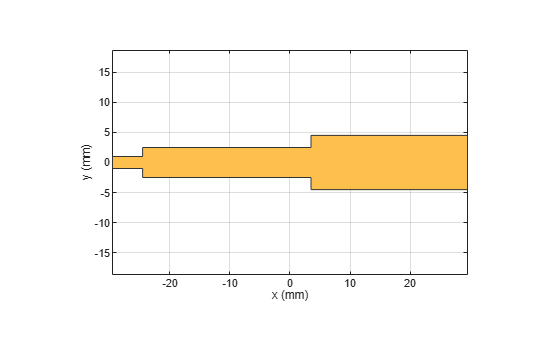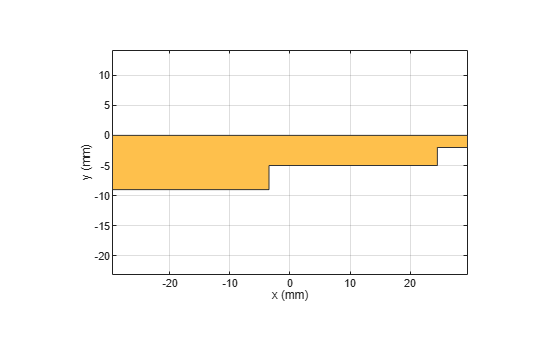# traceStep

Create step trace in XY plane

## Description

Use the `traceStep` object to create a step trace in the XY plane.

## Creation

### Syntax

``trace = traceStep``
``trace = traceStep(Name=Value)``

### Description

example

````trace = traceStep` creates a step trace in the XY plane.```
````trace = traceStep(Name=Value)` sets Properties using one or more name-value arguments. For example, `traceStep(ReferencePoint=[1 1])` creates a step trace with the reference point `[1 1]`. Properties not specified retain their default values.```

## Properties

expand all

Name of the step trace, specified as a character vector or a string scalar.

Example: `trace = traceStep(Name="traceStep")`

Data Types: `char` | `string`

X and Y coordinate of the step trace in meters, specified as a two-element vector of real elements.

Example: `trace = traceStep(ReferencePoint=[1 1])`

Data Types: `double`

Length of each section of the step trace in meters, specified as a vector of positive elements.

Example: `trace = traceStep(Length=[0.0300 0.0200 0.0230])`

Data Types: `double`

Width of each section of the step trace in meters, specified as a vector of positive elements.

Example: `trace = traceStep(Width=[0.0060 0.0060 0.0080])`

Data Types: `double`

Symmetry of the step trace along the X-axis, specified as `1` or `0`. If set to `1`, symmetry is enabled. Set the property to 1 to create a symmetric step trace and to 0 to create a nonsymmetric step trace.

Example: `trace = traceStep(Symmetry=0)`

Data Types: `logical`

## Object Functions

 `add` Boolean unite operation on two RF PCB shapes `subtract` Boolean subtraction operation on two RF PCB shapes `intersect` Boolean intersection operation on two RF PCB shapes `plus` Shape1 + Shape2 for RF PCB shapes `minus` Shape1 - Shape2 for RF PCB shapes `and` Shape1 & Shape2 for RF PCB shapes `area` Calculate area of RF PCB shape in square meters `rotate` Rotate RF PCB shape about defined axis `rotateX` Rotate RF PCB shape about x-axis `rotateY` Rotate RF PCB shape about y-axis and angle `rotateZ` Rotate RF PCB shape about z-axis `translate` Move RF PCB shape to new location `scale` Change size of RF PCB shape by fixed amount

## Examples

collapse all

Create a step trace with default values.

`trace = traceStep`
```trace = traceStep with properties: Name: 'mytraceStep' ReferencePoint: [0 0] Length: [0.0050 0.0280 0.0260] Width: [0.0020 0.0050 0.0090] Symmetry: 1 ```

Visualize the step trace.

`show(trace)`Create a nonsymmetrical stepped trace.

```trace = traceStep; trace.Symmetry = 0;```

Rotate the step trace by 180 degrees about the Z-axis.

`trace = rotateZ(trace,180);`

Visualize the step trace.

`show(trace)`## Version History

Introduced in R2022a